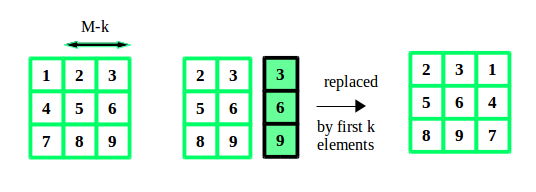Open In App

# C++ Program to Rotate the matrix right by K times

Given a matrix of size N*M, and a number K. We have to rotate the matrix K times to the right side.
Examples:

```Input :  N = 3, M = 3, K = 2
12 23 34
45 56 67
78 89 91

Output : 23 34 12
56 67 45
89 91 78

Input :  N = 2, M = 2, K = 2
1 2
3 4

Output : 1 2
3 4```

A simple yet effective approach is to consider each row of the matrix as an array and perform an array rotation. This can be done by copying the elements from K to end of array to starting of array using temporary array. And then the remaining elements from start to K-1 to end of the array.
Lets take an example:## C++

 `// CPP program to rotate a matrix right by k times``#include ` `// size of matrix``#define M 3``#define N 3` `using` `namespace` `std;` `// function to rotate matrix by k times``void` `rotateMatrix(``int` `matrix[][M], ``int` `k) {``  ``// temporary array of size M``  ``int` `temp[M];` `  ``// within the size of matrix``  ``k = k % M;` `  ``for` `(``int` `i = 0; i < N; i++) {` `    ``// copy first M-k elements to temporary array``    ``for` `(``int` `t = 0; t < M - k; t++)``      ``temp[t] = matrix[i][t];` `    ``// copy the elements from k to end to starting``    ``for` `(``int` `j = M - k; j < M; j++)``      ``matrix[i][j - M + k] = matrix[i][j];` `    ``// copy elements from temporary array to end``    ``for` `(``int` `j = k; j < M; j++)``      ``matrix[i][j] = temp[j - k];``  ``}``}` `// function to display the matrix``void` `displayMatrix(``int` `matrix[][M]) {``  ``for` `(``int` `i = 0; i < N; i++) {``    ``for` `(``int` `j = 0; j < M; j++)``      ``cout << matrix[i][j] << ``" "``;``    ``cout << endl;``  ``}``}` `// Driver's code``int` `main() {``  ``int` `matrix[N][M] = {{12, 23, 34},``                     ``{45, 56, 67},``                     ``{78, 89, 91}};``  ``int` `k = 2;` `  ``// rotate matrix by k``  ``rotateMatrix(matrix, k);` `  ``// display rotated matrix``  ``displayMatrix(matrix);` `  ``return` `0;``}`

Output:

```23 34 12
56 67 45
89 91 78```

Time Complexity: O(N*M)
Auxiliary Space: O(M)

Please refer complete article on Rotate the matrix right by K times for more details!